# CLIJ2GPU accelerated image processing for everyone

CLIJ2 home

# Filtering in graphs

Authors: Robert Haase, Daniela Vorkel, April 2020

Source

This macro shows how to apply a filter to values of a graph.

```
// initialize GPU
run("CLIJ2 Macro Extensions", "cl_device=[GeForce RTX 2060 SUPER]");
Ext.CLIJ2_clear();

run("Close All");

```

## Define a list of point coordinates and push them to the GPU

```
// make a pointlist of random coordinates in the GPU
number_of_points = 100;
number_of_dimensions = 2;
bit_depth = 32;
Ext.CLIJ2_create2D(pointlist, number_of_points, number_of_dimensions, bit_depth);
random_min = 0;
random_max = 100;
seed = getTime();
Ext.CLIJ2_setRandom(pointlist, random_min, random_max, seed);
Ext.CLIJ2_pull(pointlist);
zoom(10);

```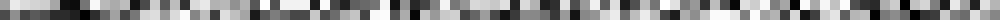## Draw labelled spots (1, 2, 3, …) in 2D space

```Ext.CLIJ2_pointlistToLabelledSpots(pointlist, spots_image);
Ext.CLIJ2_pull(spots_image);
zoom(4);
run("glasbey_on_dark");

```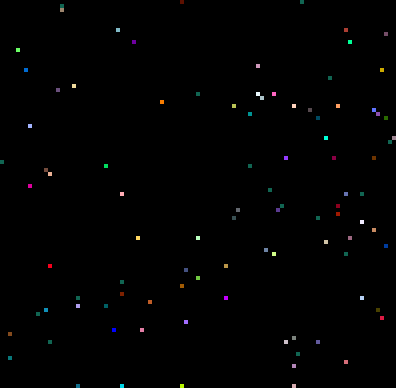## Partition the space between points

```Ext.CLIJ2_labelVoronoiOctagon(spots_image, labelled_voronoi);
Ext.CLIJ2_pull(labelled_voronoi);
zoom(4);
run("glasbey_on_dark");;

```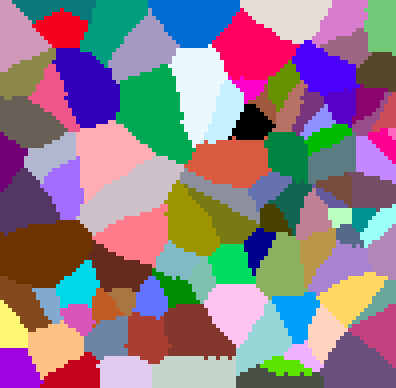## Distribute random measurements in space - with one outlier

```measurement_array = newArray(number_of_points);
for (i = 0; i < number_of_points; i += 1) {
measurement_array[i] = random() * 10;
}
measurement_array[number_of_points / 2] = 20;

Ext.CLIJ2_pushArray(measurement, measurement_array, number_of_points, 1, 1);
Ext.CLIJ2_pull(measurement);
zoom(10);

```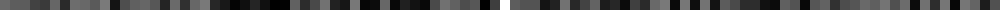## Create a parametric image showing measurements in 2D space

```Ext.CLIJ2_replaceIntensities(labelled_voronoi, measurement, parametric_image);
Ext.CLIJ2_pull(parametric_image);
zoom(4);
setMinAndMax(0, 20);
run("Fire");

```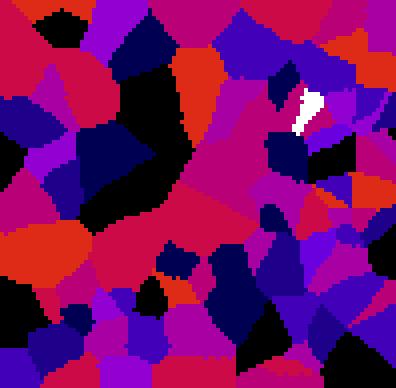## Calculate the median of every node and its neighbors in the graph

```
// determine the touch matrix
Ext.CLIJ2_generateTouchMatrix(labelled_voronoi, touch_matrix);

/// determine the median value of each neighboring nodes
Ext.CLIJ2_medianOfTouchingNeighbors(measurement, touch_matrix, median_measurement);

// draw measurements of the median, again as parametric image
Ext.CLIJ2_replaceIntensities(labelled_voronoi, median_measurement, parametric_image);
Ext.CLIJ2_pull(parametric_image);
zoom(4);
setMinAndMax(0, 20);
run("Fire");

```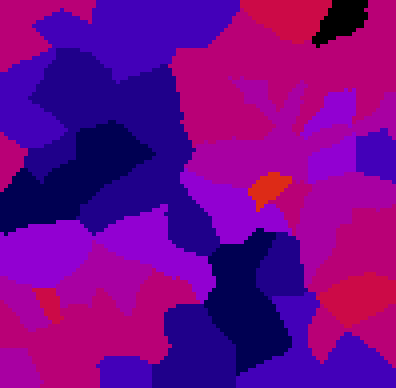As you can see, the outlier is gone! :-)

```
```

At the end of the macro, clean up:

```Ext.CLIJ2_clear();

```

This is just a useful function to get a nice visualization in the notebook:

```function zoom(factor) {
getDimensions(width, height, channels, slices, frames);
before = getTitle();
run("Scale...", "x=" + factor + " y=" + factor + " width=" + (width * factor) + " height=" + (height* factor) + " interpolation=None average create");
selectWindow(before);
close();
}

```